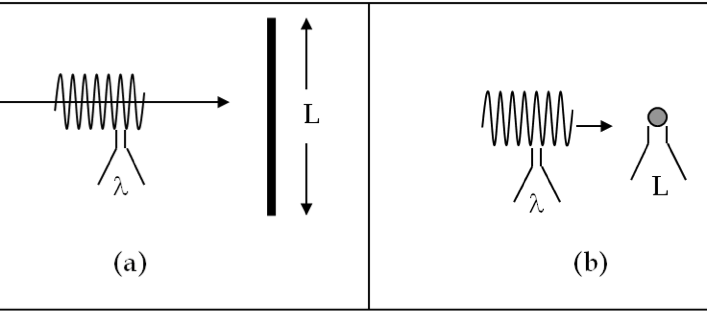## Section46.2Ray Optics versus Wave Optics

What happens when an electromagnetic wave encounters an object? That depends on the relative size of the object and wavelength of light. We will denote the size of the object by its smalles dimension along any direction by letter $L\text{.}$ We assume that $L$ is not atomic size so that we can treat everything classically.

The interaction between light particles and matter particles occur through the electric charge of the matter particles and electric and magnetic fields associated with the electromagnetic wave of light. Upon interaction, matter reradiates light - the new light back into the original medium is called reflected light and that goes inside the matter is called transmitted or refracted light.

Although, we could study the classical interaction between light and matter in terms of wave picture of light, these details are not necessary in many practical situations, such as studying microscopes and telescopes. We distinguish two types of analysis as outlined below and illustrated in Figure 46.2.1.

Ray/Geometric Optics: If the wavelength $\lambda$ of light is much smaller than the size $L$ of the object and we are only interested in directions of reflected and transmitted lights, then, we could treat light beam simply by a ray picture. When we do that, we say that we are doing geometric or ray optics.

Wave/Physical Optics: However, if $\lambda \ge L$ or $L$ only a few times $\lambda$ so that we cannot claim $\lambda \lt \lt \text{,}$ then ray picture will give us misleading answers. In this situation, we must use the wave picture and treat light as electromagnetic wave. Also, if we are interested in how much energy in the light ends up in the reflected part and how much in the transmitted part, we must use the wave picture. Physical optics is another term for wave optics.Figure 46.2.1. (a) Geometric optics situation applies when wavelength is much smaller than the dimensions of objects the light interacts with, and (b) Physical or wave optics situation applies when the wavelength of the light is of the same order as the dimension of the object.

Although geometrical optics can also be understood from the more fundamental subject of physical optics, it is far easier to apply, and when applicable, it is definitely preferred. Thus, when the red light of wavelength 700 nm strikes a lens of size 1 cm it is best to use rules of geometrical optics to figure out what will happen, but when the same light strikes a thin fiber of diameter $1\ \mu\text{m}$ you will have to use physical optics to understand what is going on.

Decide when you will have to use wave optics in the following situations.

(a) A green laser light of wavelength $530\text{ nm}$ is incident on a mirror of size $30\text{ cm}\text{.}$ Will your answer change if you also needed to fidn intensity of the reflected and transmitted waves?

(b) A red laser light of wavelength $632\text{ nm}$ is incident on a bead of diameter $10\:\mu\text{m}\text{.}$ Will your answer change if you also needed to fidn intensity of the reflected and transmitted waves?

(c) A radio wave of frequency $10^9\text{ Hz}$ is incident on a square aluminum plate of area $9 \text{m}^2\text{.}$ Will your answer change if you also needed to fidn intensity of the reflected and transmitted waves?

(d) A radio wave of frequency 10^9\text{ Hz} incident on a square aluminum plate of area $9\text{ cm}^2\text{.}$ Will your answer change if you also needed to fidn intensity of the reflected and transmitted waves?

Hint

Compare wavelength with the dimension of the object.

(a) Ray, (b) Wave, (c) Ray, (d) Wave. If intensity required, we need to study wave picture.

Solution 1 (a)

(a) Here dimension is $L=30\text{ cm}$ and wavelength $\lambda = 530\text{ nm}\text{.}$ These give us

\begin{equation*} \frac{L}{\lambda} = 5.66\times 10^5. \end{equation*}

That is $L \gt\gt \lambda\text{.}$ We will use ray optics here if we do not need to study intensity.

Yes, the answer will change since for intensity studies we need the wave picture.

Solution 2 (b)

(b) Here dimension is $L=2.0\:\mu\text{m}$ and wavelength $\lambda = 632\text{ nm}\text{.}$ These give us

\begin{equation*} \frac{L}{\lambda} = 3.16. \end{equation*}

That is $L \sim \lambda\text{.}$ We must use wave optics here.

No, the answer will not change since for intensity studies we need the wave picture.

Solution 3 (c)

(c) Compare $\lambda$ to the smallest dimensions of the plate, which would be the square-root of the area, $\sqrt{A}\text{.}$ Note, we cannot compare a length to an area, that is why we must get the square root of the area. Here

\begin{align*} \lambda \amp = \frac{3\times 10^8\:\textrm{m/s}}{10^9\:\textrm{Hz}} = 0.3\:\textrm{m}\\ sqrt{A} = 3 \text{ m} \end{align*}

Therefore, we have $\lambda \lt \lt \sqrt{A}\text{,}$ we can use the ray approximation if we do not need intensity studies.

Yes, the answer will change since for intensity studies we need the wave picture.

Solution 4 (d)

(d) Here, $\sqrt{A}= 0.03\text{ m}$ and $\lambda = 0.3\text{ m}\text{.}$ Since $\lambda \gt \gt \sqrt{A}\text{,}$ we need to use the wave picture.

No, the answer will not change since for intensity studies we need the wave picture.